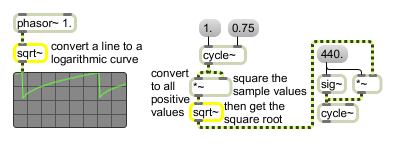# sqrt~

Square root of a signal

## Description

sqrt~ will output a signal which is the square-root function of the input signal.

None.

## Messages

 signal The object outputs a signal that is the square root of the input signal. A negative input has no real solution, so it causes an output of 0.

## Output

signal: The square root of the input signal.

## ExamplesOutput signal is the square root of the input signal